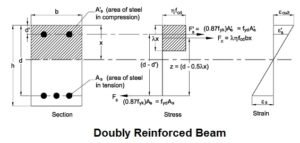# Doubly Reinforced Beam

## Doubly Reinforced Beam:R.C.C beam which comprises of reinforcement both in tension zone, as well as compression zone is called doubly reinforced beam.

Doubly reinforced beam is generally adopted in following conditions:

1. When the size of the beam is confined.
2. When the section of the beam is subjected to inversion stress.
3. When the beam is nonstop more than a few backings.

Critical NA of a doubly reinforced beam is calculated by this given formula:

n = mcd/t+mc

Where, n = Critical NA

m = Modular ratio.

c = Max. compressive stress in the concrete.

READ -  Types Of Failures In Beam

d = Effective depth of the beam.

t = Allowable tensile stress in steel.

Actual NA is determined by taking moments of the effective zone about the centroid of the effective segment.

bn2/2 + (1.5m-1)Ac(n-dc) = mAt(d-n)

Where, b = Breadth of the beam.

Ac = Area of compressive steel.

dc= Centre of gravity (c.g) of compressive region of steel from external fibres.

At = Area of tensile steel.

Doubly reinforced beam is inefficient in steel, as steel is utilized as a part of compression zone is never stressed to its full limit. Compressive stress in steel relies on the compressive stress in concrete at that level. Stress in concrete (C1)  at level of compressive steel can be determined by using following equation.

C1 = c(n-dc)/n

Moment Of Resistance In Doubly Reinforced Beam:

The total moment of resistance is calculated by adding the moments of following two couples.

1. Couple (M1) having tensile steel A and compressive concrete.

2. Couple (M2) having extra tensile steel At and compressive steel.

The moment of resistance M = M1 + M2 = (bnc/2)(d-n/3) + (1.5-1)AcC1(d-dc)

Where C1 = c(n-dc/n)